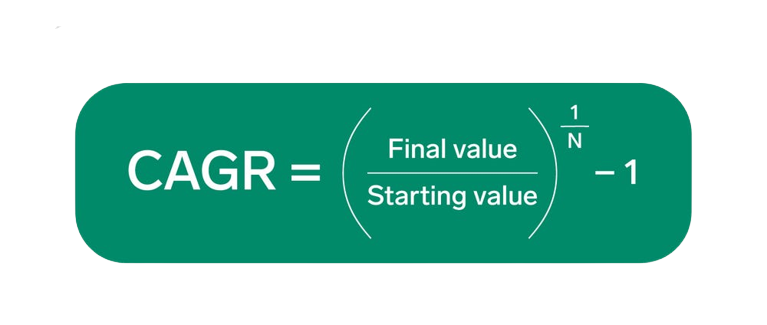CAGR CalculatorCalculate your Compound Annual Groth Rate(CAGR) Easily and quickly with all in one CAGR Calculators

EXPLORE PRODUCTS

CAGR stands for Compound Annual Growth Rate. It is a financial measure used to calculate the average annual growth rate of an investment or business over a specific period of time, assuming that the growth rate remains constant. CAGR is often used to analyze the performance of investments or evaluate the growth of a company.

The CAGR formula is:

CAGR = (Ending Value / Beginning Value)^(1 / Number of Years) - 1CAGR = (Ending Value / Beginning Value)^(1 / Number of Years) - 1

In this formula, the "Ending Value" represents the value of the investment or business at the end of the period, the "Beginning Value" represents the initial value at the beginning of the period, and the "Number of Years" is the duration of the period.

CAGR provides a standardized measure to compare the growth rates of different investments or businesses over various time frames. It smooths out the volatility and fluctuations in growth rates by providing a single, annualized growth rate that represents the overall performance of the investment or business.

A CAGR calculator helps you in several ways:

Assessing Investment Performance: If you have made investments, such as in stocks, mutual funds, or other financial instruments, a CAGR calculator can help you evaluate the historical performance of your investment. By inputting the initial investment value and the final value over a specific time period, the calculator calculates the CAGR for you. This allows you to determine the average annual growth rate of your investment. You can compare the CAGR with other investments or benchmarks to assess how well your investment has performed over time.

Projecting Future Growth: A CAGR calculator can also assist in projecting future growth based on historical performance. By inputting the current value of an investment and the desired time period, the calculator estimates the potential future value based on the average annual growth rate (CAGR) observed in the past. This can be helpful for financial planning, setting investment goals, or estimating the growth potential of a business.

Comparing Investments or Businesses: CAGR calculators are valuable tools for benchmarking and comparing the performance of investments or businesses. By calculating the CAGR for multiple investments or companies over the same time period, you can objectively compare their growth rates. This allows you to identify top-performing investments or assess how well a company has grown relative to its competitors.

Decision Making: The CAGR calculation provides a consistent measure of growth, enabling you to make informed decisions. For example, if you are considering investing in a particular stock or business, knowing its historical CAGR can help you evaluate its growth potential and make a more informed investment decision. It can also be used to compare investment opportunities and choose the one with the higher CAGR.

Overall, a CAGR calculator helps you analyze historical performance, project future growth, benchmark investments or businesses, and make data-driven decisions in various financial and business contexts. It provides a standardized metric to assess growth rates and allows you to evaluate investments or businesses more effectively.

### How to Calculate CAGR?

Here's a simplified way to calculate CAGR:

• Determine the Beginning Value (BV): Identify the initial value or investment amount at the start of the period.
• Determine the Ending Value (EV): Identify the final value or investment amount at the end of the period.
• Determine the Number of Years (N): Calculate the number of years that have elapsed between the beginning and ending values.
• Use the CAGR formula: Divide the Ending Value (EV) by the Beginning Value (BV) to get the ratio. Then, raise this ratio to the power of 1 divided by the Number of Years (N). Subtract 1 from the result.

CAGR = (EV / BV)^(1 / N) - 1

That's it! You have now calculated the CAGR, which represents the average annual growth rate of the investment or value over the specified period.

Let's calculate the CAGR using an example:

Suppose you invested \$10,000 in a stock five years ago, and now your investment is worth \$15,000. You want to calculate the CAGR over this five-year period.

• Beginning Value (BV) = \$10,000
• Ending Value (EV) = \$15,000
• Number of Years (N) = 5

Using the CAGR formula:

CAGR = (EV / BV)^(1 / N) - 1

Plug in the values:

CAGR = (\$15,000 / \$10,000)^(1 / 5) - 1

Calculating the values within the parentheses first:

CAGR = (1.5)^(1 / 5) - 1

Now, calculate the exponent:

CAGR = 1.086 - 1

Finally, subtract 1 from the result:

CAGR = 0.086 or 8.6%

Therefore, the CAGR for your investment over the five-year period is approximately 8.6%. This means, on average, your investment grew by 8.6% each year during this time, assuming the growth rate remained constant.